Note - Jeff Kinne’s version of this file, with some parts complete.

### Goals:

1. Explore the structure of the data
2. Assess the quality of the data
3. Identify metabolites that are different between cancer types

### Requirements:

1. R or Rstudio
2. Packages:
• ggplot2 (to install, type “install.packages(”ggplot2“)” in R)
• pheatmap (to install, type “pheatmap (”ggplot2“)” in R)
• RColorBrewer (to install, type “RColorBrewer (”ggplot2“)” in R)

### Input Data:

Users can load an Rdata frame which contains two R objects:

1. metab: a matrix containing metabolite abundance values.
2. sampannot: annotation of the samples where the column “name” matches that of the metab matrix.

### Tips:

• If you forget how a function is used in R, type “?function” to get more information
• The data in metab contains replicates
• To install R packages, it’s easiest not to be on the OSUMC network.

### 1. Explore the structure of the data

``````  setwd("~jkinne/public_html/bd4isu-summer-2019/code/")
``````##           (p-Hydroxyphenyl)lactic acid 3-phospho-d-glycerate
## META-7889                        0.072                 0.927
## META-7890                        0.072                 1.389
## META-7891                        0.072                 1.695
## META-7892                        0.483                 1.322
## META-7893                        1.000                 1.883
## META-7894                        0.507                 0.118
##           5,6-Dimethylbenzimidazole 5-oxoproline
## META-7889                     0.441        1.002
## META-7890                     0.542        1.436
## META-7891                     0.685        1.249
## META-7892                     0.064        0.392
## META-7893                     0.064        0.571
## META-7894                     0.064        0.476``````
``  head(sampannot)``
``````##        name cancertype cell_line
## 1 META-7889     Breast    BT-549
## 2 META-7890     Breast    BT-549
## 3 META-7891     Breast    BT-549
## 4 META-7892     Breast   HS 578T
## 5 META-7893     Breast   HS 578T
## 6 META-7894     Breast   HS 578T``````

QUESTIONS: Using functions such as “dim”, “nrow”,“ncol”, “colnames”, “rownames”, answer the following questions:

1. How many metabolites are there?
2. How many samples are there?
3. How many cell lines are there?
4. How many cancer types are there?
5. How many replicates per cell line are there?
``````# 1.
num_metabs <- ncol(metab)
print(paste("Number of metabolites:", num_metabs))``````
``##  "Number of metabolites: 352"``
``````#2.
num_samples <- nrow(metab)
print(paste("Number of samples:", num_samples))``````
``##  "Number of samples: 39"``
``````#3.
num_cell_lines <- length(unique(sampannot\$cell_line))
print(paste("Number of cell lines:", num_cell_lines))``````
``##  "Number of cell lines: 13"``
``````#4.
num_cancer_types <- length(unique(sampannot\$cancertype))
print(paste("Number of cancer types:", num_cancer_types))``````
``##  "Number of cancer types: 2"``
``````#5.
t <- table(sampannot\$cell_line)
print(paste("Number of replicates per cell ranges in:", range(t)))``````
``````##  "Number of replicates per cell ranges in: 3"
##  "Number of replicates per cell ranges in: 3"``````

** Assess the presence of missing/imputed values. In this dataset, missing values are imputed by the minimum. One quick way to check for missing values, is to count the number of times the minumum abundance value appears for a given metabolite. One could also calculate the standard deviation and perhaps filter out metabolites with low variation. Let’s do the latter here. (If you have time on your hands, do both!) **

``````    sds=as.numeric(apply(metab,2,sd))

# Now plot the distribution of standard deviations:
hist(sds,breaks=1000,main="Distribution of metabolite abundance sds")``````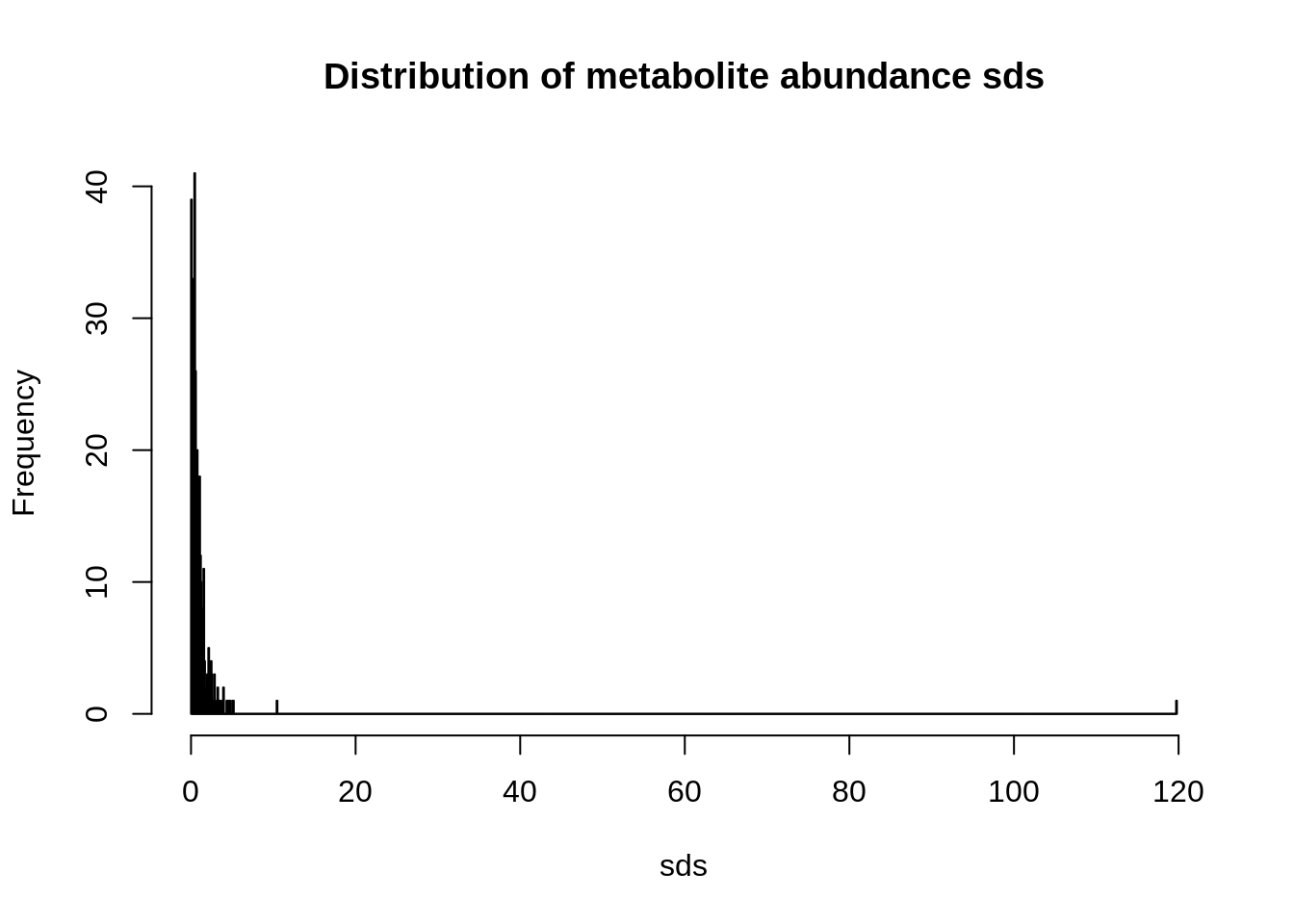Do you notice any outliers? Let’s zoom in a little:

``    hist(sds,breaks=1000,main="Distribution of metabolite abundance sds",xlim=c(0,10))``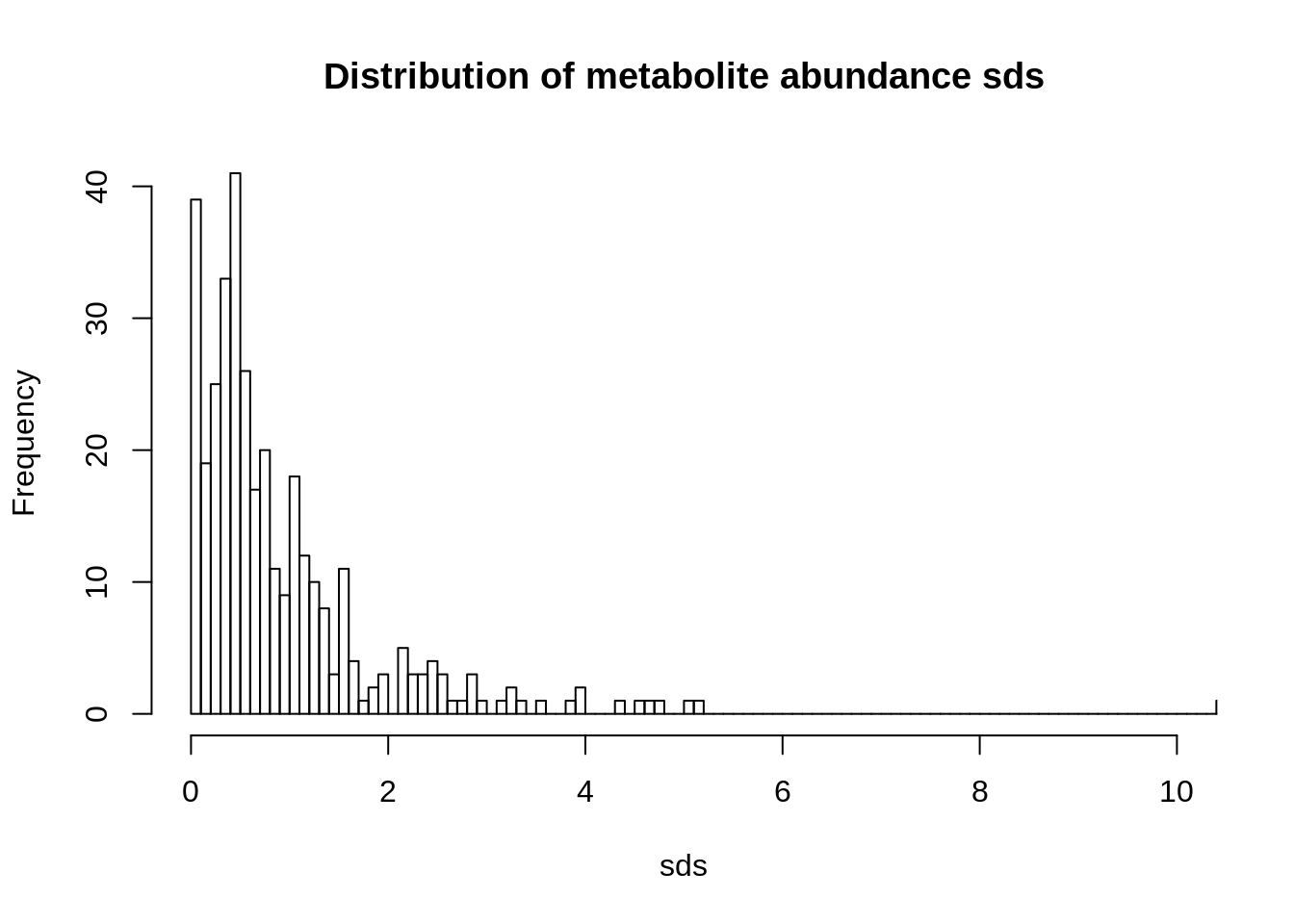Do you notice more outliers? What metabolites could you remove from further analysis? How many are there? What metabolite has a very high standard deviation?

``````    bads=c(which(sds==0),which(sds>15))
``##  34``
``    hist(sds[-bads])``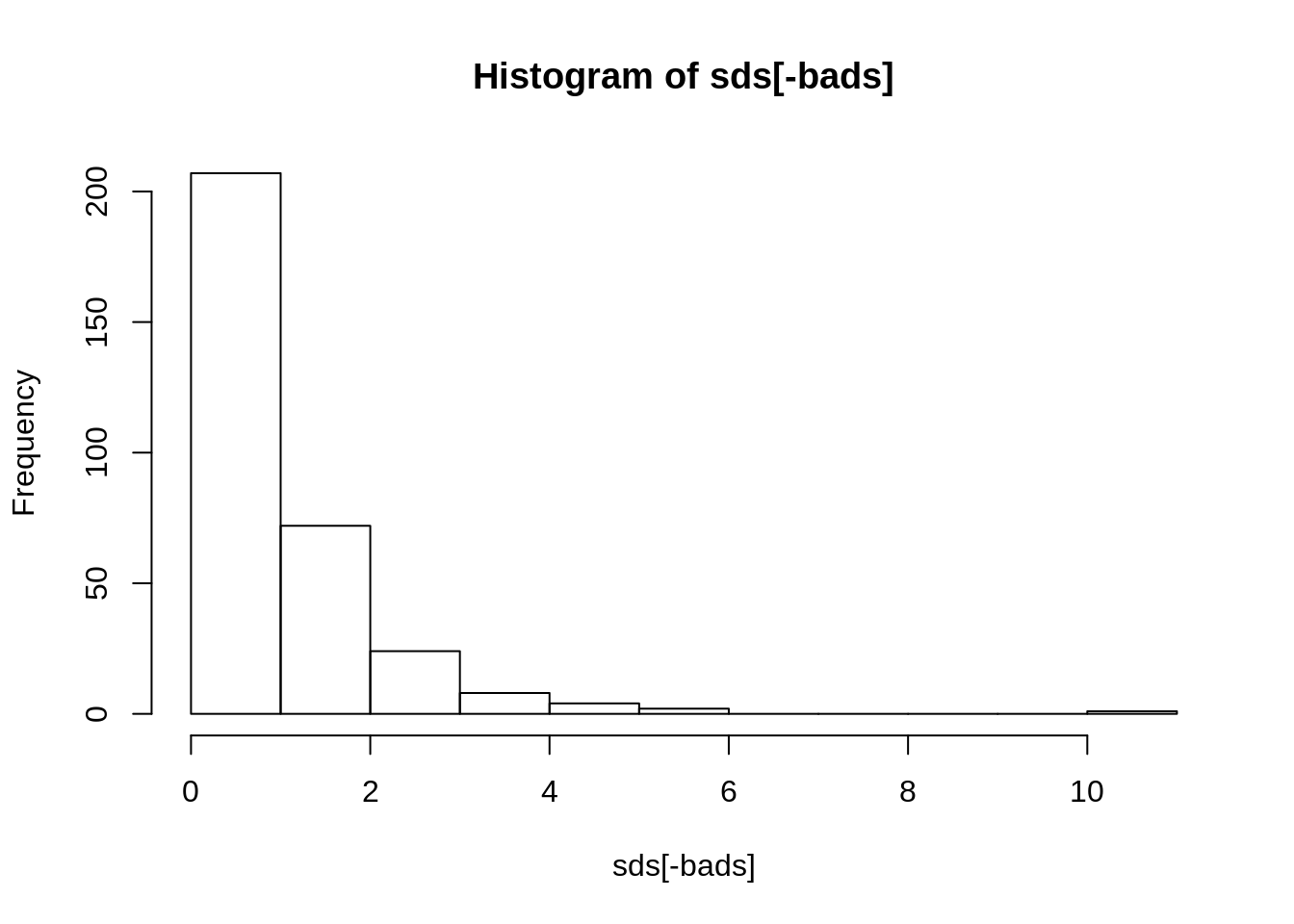``    colnames(metab)[which(sds>=10)]``
``##  "gluconic acid" "sorbitol"``
``````    # Save original and filter out metabolites
metab_o=metab

### 2. Assess the quality of the data

Look at the distsribution of metabolite abundances across each sample using a simple boxplot:

``    boxplot(as.data.frame(t(metab)),pch=19)``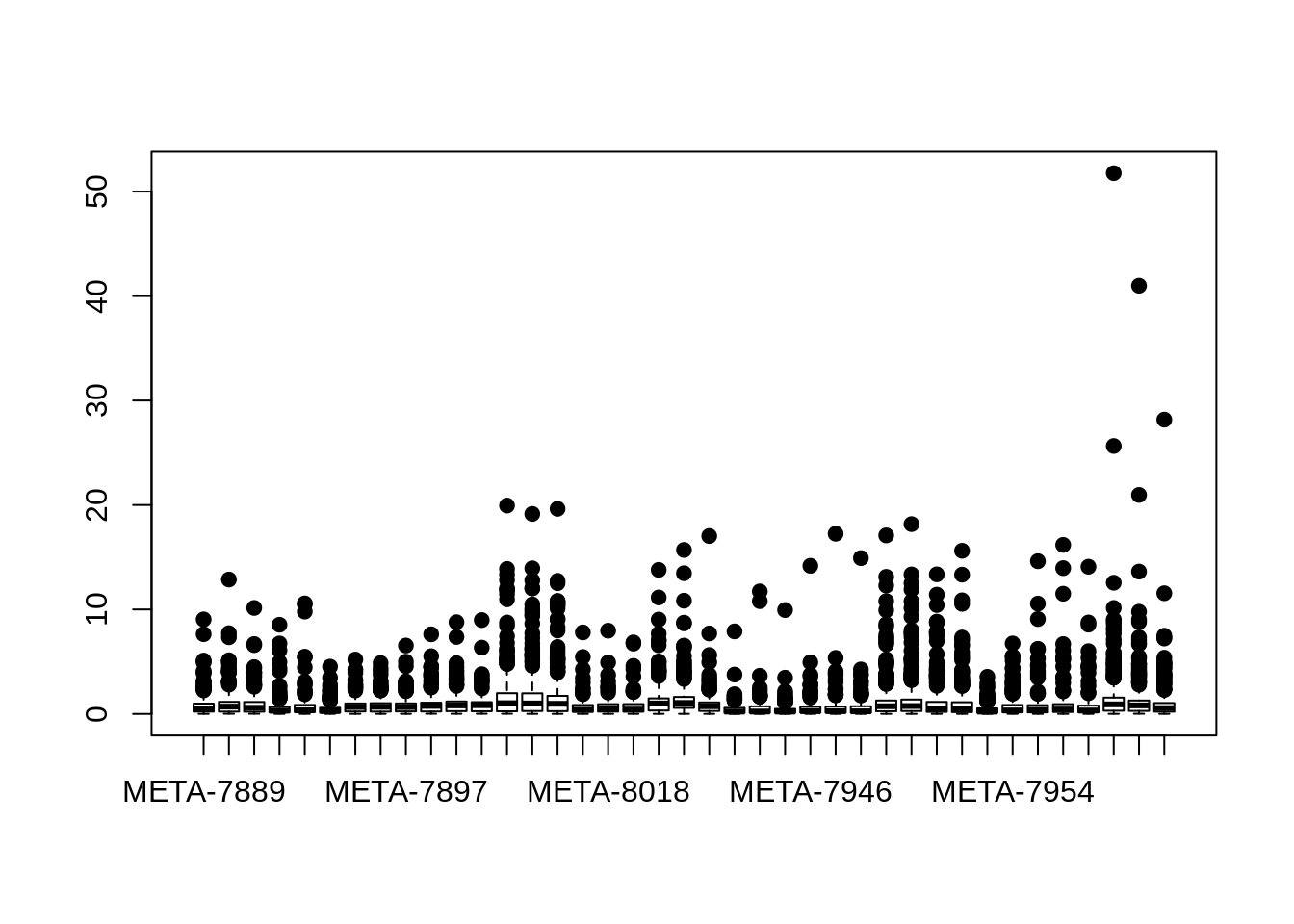Does the data need to be transformed? Try this:

``````  mycol=c(rep("slategrey",3),rep("lightsteelblue1",3),rep("lightsteelblue3",3),
rep("seagreen4",3),rep("lightskyblue",3),rep("grey",3),rep("skyblue3",3),
rep("lightcoral",3),rep("indianred4",3),rep("lightsalmon3",3),rep("salmon",3),
rep("sandybrown",3),rep("tomato",3))

boxplot(as.data.frame(t(log2(metab))),pch=19,
main="Distribution of Metabolites Per Sample",names=F,col=mycol,
ylab="log2(Normalized metabolite abundances)")

text(x =  seq_along(rownames(metab)), y=par("usr") - 0.5, srt = 45, adj = 1,
labels = rownames(metab), xpd = TRUE, cex=0.6)

legend(3,8,legend=c("Blues: breast cancer","Reds: leukemias"))``````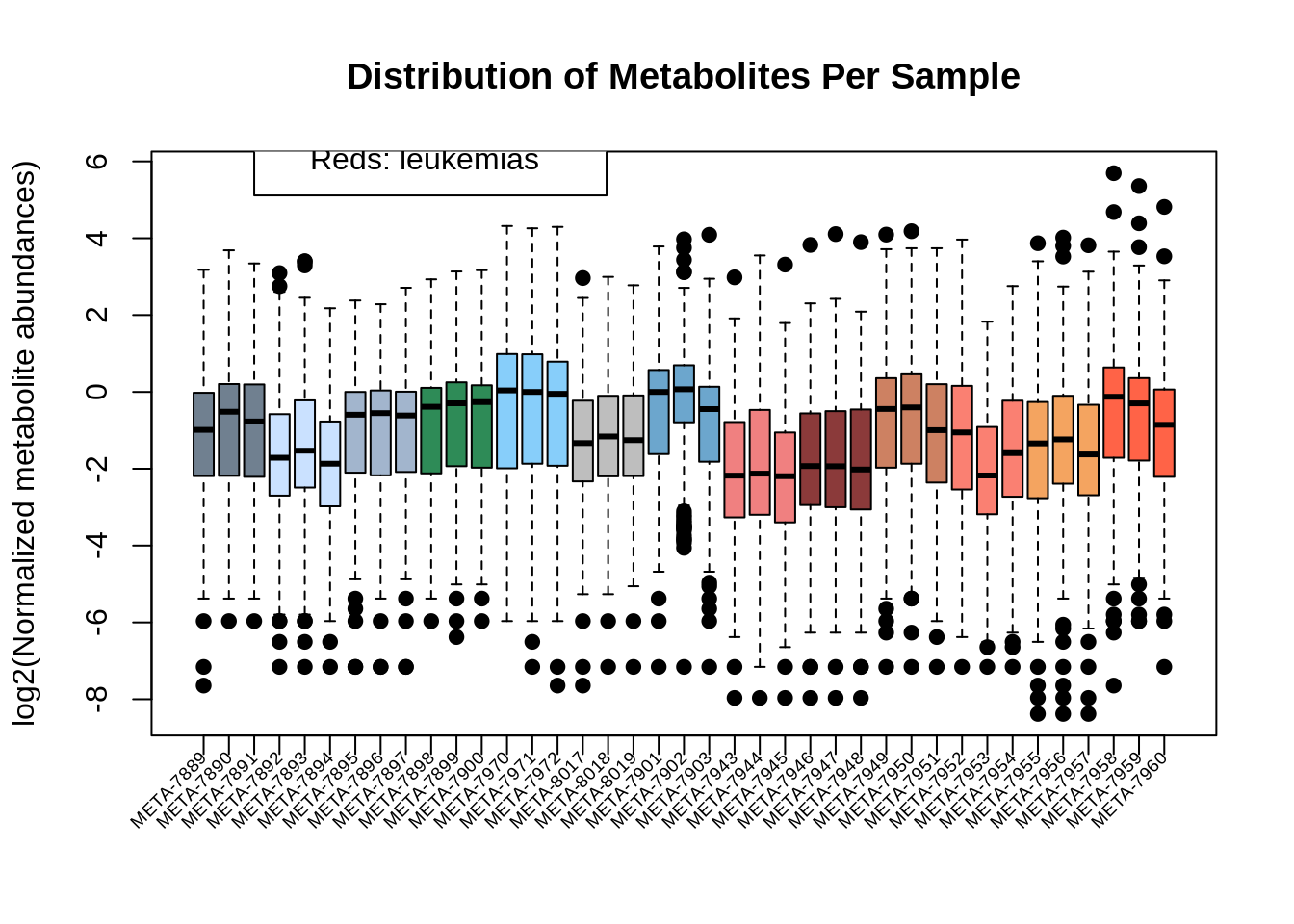Apply an unsupervised clustering method to see how samples cluster together.
For example, using PCA
:

``````  library(ggplot2)  # I assume ggplot2 is installed, if not, see above
mypca=prcomp(log2(metab),center=F,scale=T)
percvar=round((mypca\$sdev)^2 / sum(mypca\$sdev^2)*100,2)
# Check that order of mypca matrix is same as sample matrix:
all.equal(rownames(mypca\$x),rownames(metab))``````
``##  TRUE``
``````    mydf=data.frame(PC1=mypca\$x[,"PC1"],PC2=mypca\$x[,"PC2"],
Cell_Line=sampannot\$cell_line, Cancer_Type=sampannot\$cancertype)

ggplot(mydf,aes(PC1,PC2,color=Cell_Line,shape=Cancer_Type)) +
geom_point(size=7) +
scale_color_manual(values=c("slategrey","lightsteelblue1","lightsteelblue3",
"seagreen4","lightskyblue","grey","skyblue3","lightcoral","indianred4",
"lightsalmon3","salmon","sandybrown","tomato")) +
xlab(paste0("PC1: ",percvar,"% variance")) +
ylab(paste0("PC2: ",percvar,"% variance")) +
theme_bw() +
ggtitle("Metabolomics PCA Plot \n log2(normalized values)") +
theme(axis.line = element_line(colour = "black"),
axis.title=element_text(size=15,face="bold"),
plot.title=element_text(size=20,face="bold"),
panel.grid.major = element_blank(),
panel.grid.minor = element_blank(),
panel.background = element_blank(),
legend.key=element_blank())``````